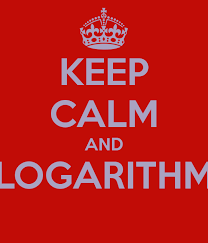# Logressions

Algebra Level 3$x,y,z$ are real numbers that satisfy the equation below

$\large \log (x-2y+z)+\log (x+z)=2\log (x-z)$

What kind of progression do $x,y,z$ form?

Details and Assumptions

• $AP$ denotes Arithmetic Progression

• $GP$ denotes Geometric Progression

• $HP$ denotes Harmonic Progression

• $AGP$ denotes Arithmetic-Geometric Progression

×

Problem Loading...

Note Loading...

Set Loading...